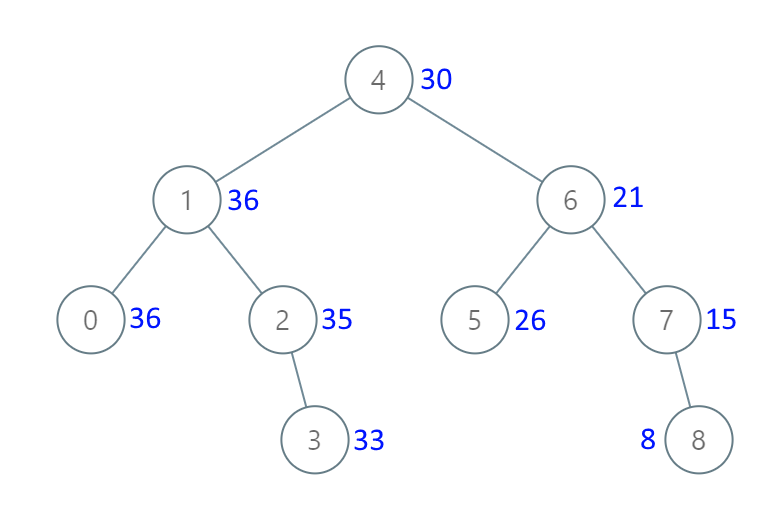## Algorithm

Problem Name: 538. Convert BST to Greater Tree

Given the `root` of a Binary Search Tree (BST), convert it to a Greater Tree such that every key of the original BST is changed to the original key plus the sum of all keys greater than the original key in BST.

As a reminder, a binary search tree is a tree that satisfies these constraints:

• The left subtree of a node contains only nodes with keys less than the node's key.
• The right subtree of a node contains only nodes with keys greater than the node's key.
• Both the left and right subtrees must also be binary search trees.

Example 1:```Input: root = [4,1,6,0,2,5,7,null,null,null,3,null,null,null,8]
Output: [30,36,21,36,35,26,15,null,null,null,33,null,null,null,8]
```

Example 2:

```Input: root = [0,null,1]
Output: [1,null,1]
```

Constraints:

• The number of nodes in the tree is in the range `[0, 104]`.
• `-104 <= Node.val <= 104`
• All the values in the tree are unique.
• `root` is guaranteed to be a valid binary search tree.

## Code Examples

### #1 Code Example with C++ Programming

```Code - C++ Programming```

``````
class Solution {
public:
TreeNode* convertBST(TreeNode* root) {
int sum = 0;
DFS(root, sum);
return root;
}

void DFS(TreeNode* root, int& sum){
if(!root) return;
DFS(root->right, sum);
sum = (root->val += sum);
DFS(root->left, sum);
}
};
``````
Copy The Code &

Input

cmd
root = [4,1,6,0,2,5,7,null,null,null,3,null,null,null,8]

Output

cmd
[30,36,21,36,35,26,15,null,null,null,33,null,null,null,8]

### #2 Code Example with Javascript Programming

```Code - Javascript Programming```

``````
const convertBST = function(root) {
const arr = []
traverse(root, arr)
for(let i = arr.length - 2; i >= 0; i--) {
arr[i].val += arr[i + 1].val
}
return root
};

function traverse(node, arr) {
if(node == null) return
traverse(node.right, arr)
arr.unshift(node)
traverse(node.left, arr)
}
``````
Copy The Code &

Input

cmd
root = [4,1,6,0,2,5,7,null,null,null,3,null,null,null,8]

Output

cmd
[30,36,21,36,35,26,15,null,null,null,33,null,null,null,8]

### #3 Code Example with Python Programming

```Code - Python Programming```

``````
class Solution:
def convertBST(self, root):
"""
:type root: TreeNode
:rtype: TreeNode
"""
def traverse(node):
if not node: return
traverse(node.right)
node.val = residue = node.val + residue
traverse(node.left)
return node
residue = 
return traverse(root)
``````
Copy The Code &

Input

cmd
root = [0,null,1]

Output

cmd
[1,null,1]

### #4 Code Example with C# Programming

```Code - C# Programming```

``````
namespace LeetCode
{
public class _0538_ConvertBSTToGreaterTree
{
private int sum = 0;

public TreeNode ConvertBST(TreeNode root)
{
if (root != null)
{
ConvertBST(root.right);
sum += root.val;
root.val = sum;
ConvertBST(root.left);
}

return root;
}
}
}
``````
Copy The Code &

Input

cmd
root = [0,null,1]

Output

cmd
[1,null,1]